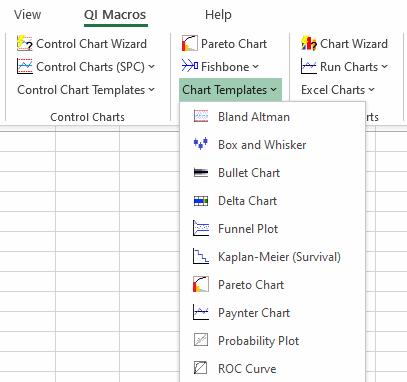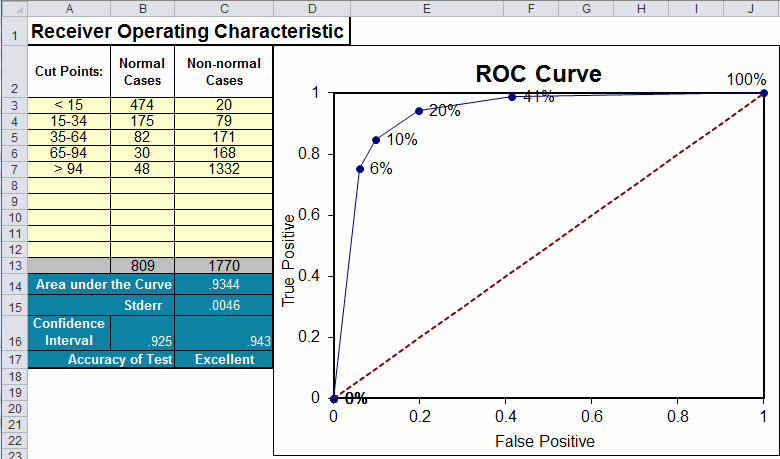# ROC Curve in Excel (Receiving Operating Characteristic)

## Use a ROC Curve to choose the most appropriate "cut-off" for a test.

### QI Macros Add-in for Excel Contains a ROC Curve Template

To open the template, click on QI Macros Menu and select, Chart Templates, ROC Curve.### To complete the ROC Curve template:

1. Input the Cut Points in column A.
2. Input the number of normal and non-normal cases in columns B and C, respectively.
3. The template will perform the calculations and draw the ROC Curve.
4. The template will also calculate the area under the curve (C14) and rate the accuracy of the test (C17).
• > .9 = Excellent
• > .8 = Good
• > .7 = Fair
• ≤ .7 = Poor

For a comprehensive description of ROC Curves see: http://gim.unmc.edu/dxtests/ROC1.htm